# Angles, and More Lines

### Sundials, Gnomons, Eclipses, and Transits

Astronomical events dictate the day, lunar month, and year. When man wanted to subdivide a day, the sundial proved useful. Here you will find geometry activities with a sundial theme. There are many variations on the sundial theme. However, one vocabulary word from this is gnomon, the part of the sundial which casts the shadow. For a horizontal sundial, what is the appropriate angle this makes with the horizon? A visit here might be useful.

Another study of angles involves eclipses and transits. The student is assumed to be familiar with such events, especially, the pair of venus transits in June 2004 and 2012. These are rare enough that no one alive has seen one.

### Basic Angles

 An angle is the union of two rays with the same endpoint.

The rays forming an angle are its sides. The vertex is the common endpoint of the two rays. The symbol for angle (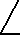) can easily be confused with the symbol for less than (<). One important classification of angles is based on their angle measure. Angles are usually measured in either degrees or radians. (See also an introduction in Numbers Lesson 11.) Occasionally we see references to grade (1 grade=0.9°). Although some (our text) omit the degree symbol, we don't expect you ever to. There are 360° or 2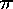radians in a complete revolution or circle. It is important to differentiate between an angle (a set of points) and its measure denoted m. A zero angle is where the two rays share the same points and coincide. They thus appear to be a single ray. It is so named because of its zero angle measure. An acute angle is one whose measure is strictly between zero and 90°. A right angle is one whose measure is exactly 90°. On a drawing the symbol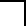can be used to label two rays or segments as perpendicular (at right angles). In symbols we write that one line or segment is perpendicular to another using the symbol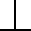. An obtuse angle is one whose measure is strictly between 90° and 180°. A straight angle is one in which both rays are opposite and form a [straight] line. Its measure is 180°. Not all geometries accept zero and straight angles as angles, often specifying that the component rays must be nonidentical and nonopposite. Two more angle terms are reflex angle for angles between 180° and 360° and full angle or 360° (one revolution). Angles can sometimes be named by their vertex alone (A), but since more than one angle often share a vertex this "nickname" can be ambiguous and a full specification using three points (BAC) is thus necessary. At other times angles may be designated via a number (see below).

### Pairs of Angles

Angles often come in pairs which might have special names depending on their relative size or location. Adjacent angles are angles which share a side or ray. A pair of complementary angles sum to 90°. A pair of supplementary angles sum to 180°. A linear pair of angles are both supplementary and adjacent. Vertical angles share a vertex and are formed by extending each ray though the vertex. The letter X provides some good examples. The angle opening upward and the angle opening downward are vertical angles as are the pair opening left and right. Vertical angles have an easily proved property given below. You can prove this property by noting that these two angles are supplementary to the same angle.

 Vertical angles are equal.

Angles separate their plane into three regions: the angle; the region inside or interior to the angle; and the region outside or exterior to the angle. For angles of measure between 0° and 180°, the interior is the convex set. A ray which extends from the vertex of an angle through the interior such that it divides the angle into two congruent (same shape and measure) angles is called an angle bisector. Bisect means to cut into two equal pieces.

### Angles in Relative Positions

Angles are also formed when a transversal intersects a pair of lines, which are not necessarily parallel. A prototypical example would be the not equal symbol: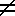. Angles which are in the same relative position but on the other line are called corresponding angles (angles 1 and 5 in the figure below right). The word alternate is often used to indicate things on opposite sides, like some leaves on a stem. In this context it means on opposite sides of the transversal. The word interior refers to the region between the lines, whereas, the word exterior refers to the regions outside the lines. We refer to those angles between the lines as interior angles (angles 3, 4, 5 and 6) and those outside the lines as exterior angles (angles 1, 2, 7 and 8). (Note also below the use of interior/ exterior angles as applied to polygons.) Thus when we have a transversal cutting two lines (not necessarily parallel) we have alternate interior angles (angles 4 and 6 below right) and alternate exterior angles (angles 1 and 7 below right). If the pair of lines are parallel, we have the following important properties.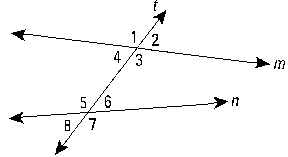Two lines cut by a transversal are parallel if and only if: Alternate interior angles are equal in measure; or Alternate exterior angles are equal in measure; or Corresponding angles are equal in measure.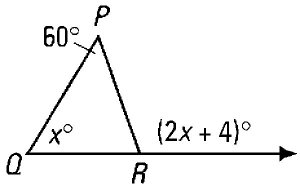The angle between two sides of a polygon is an interior angle, whereas the angle formed by one side and extending the other side of an angle in a polygon is an exterior angle. They form a linear pair. Examples of interior angles would be those labelled x and 60º in the figure left. The angle labelled (2x+4)º is an exterior angle. We can also differentiate between the interior and exterior of a polygon by noting that your right side is toward the interior as you travel clockwise around a closed figure or on your left side as you travel counter-clockwise or anti-clockwise as they say on the other side of the "pond."

### Angles from Trigonometry

There are also angles which are especially important in trigonometry. First, in trigonometry the angle vertex is placed at the origin of a coordinate system with one ray coincident with the x-axis. Angles are then measured as positive if measured counter-clockwise from the x-axis or as negative if measured clockwise from the x-axis. This is called standard position. Our geometry differentiates between rotation and angle. Thus in trigonometry angles can be more than 180° or less than 0°, whereas in geometry only rotations can be. The reference angle is then the acute (or right) angle between the ray and the x-axis. It is always between 0° and 90° inclusive. An angle in standard position is a quadrantal angle whenever its terminal side lies on an axis. Quadrantal angles are thus integer multiples of 90°. Coterminal angles are angles which share the same sides, such as 120° and -240° or 90° and 450°. Coterminal angles differ by an integral multiple of 360° or 2radians. Angles inside circles are either central angles if their vertex is the center of the circle, or inscribed angles if their vertex is on the circle. (We assume each side includes a point on the circle other than the vertex.) A central angle intercepts a portion of the circumference of the circle termed an arc. An arc is a minor arc if its central angle is less than 180°. An arc is a major arc if its central angle is more than 180°. If an arc is exactly 180° it is termed a semicircle. Minor arcs are specified using two points (letters) under a curved line, whereas semicircles and major arcs are specified using three points. There is the following relationship between a central angle and its corresponding inscribed angle.

 An inscribed angle is half the measure of the central angle intercepting the same arc.

If you know the measure of a central angle, you can also calculate its arc length as the proportion between this measure over 360° equal to this unknown arc length over the circumference.

### Assumptions: Valid and Invalid

In Geometry, we never take anything for granted, but build knowledge by proving everything step by step. This tradition dates back to the ancient Greek Pythagorean school and was codified many years later by Euclid in a 13 volume set of books called Elements. Proofs often go awry because steps are not done in a proper order or steps are omitted. Another common mistake is to make improper assumptions or not properly support assumptions. Please become familiar with the following lists!

 The following items can be assumed from figures: collinearity and betweenness of points on lines; intersection of lines at a given point; and points interior to, on, or exterior to an angle, n-gon, or circle.
 The following items cannot be assumed from figures: collinearity of points not on lines; whether or not lines are parallel or perpendicular, angles are acute or obtuse; angle measure, and segment length, especially congruence of such.

 Always remember: Geometric diagrams are not drawn to scale.

### Algebraic Properties

Although we presented many axioms about the real numbers previously in Numbers Lesson 13, we will review and summarized them again here since they apply to our geometry.
Postulates of Equalities, Inequality, and Operations:Example:
Reflexivex = x.
SymmetricIf x = y, then y = x.
TransitiveIf x = y and y = z, then x = z.
Addition Property of EqualityIf x = y, then x + z = y + z.
Multiplication Property of Equality If x = y, then x × z = y × z.
Equality to Inequality PropertyIf x and y are >0 and x+y=z, then z>x and z>y.
Substitution PropertyIf x = y, then x may be substituted for y anywhere.

An easy way to differentiate among reflexive, symmetric, and the transitive property is to relate the alphabetic sequence RST with the numeric sequence 123 and note the number of variables used in each definition. Subtraction was defined via additive inverses and division was likewise defined via multiplicative inverses (Numbers Lesson 2). Hence the Addition and Multiplication properties above allow those operations as well. Also, similar properties for inequalities (< and > but not) for the Transitive, Addition, and Multiplication (for z>0) postulates above hold as well. The next to last property given above is very similar to the Triangle Inequality. Mastering algebra is an underlying theme of geometry, as are building vocabulary, developing important concepts, and sharpening critical thinking. Graphing lines and inequalities on the coordinate plane, finding slope, determining parallelism or perpendicular, are a major venue for developing this mathematical prowess.

### Lines

We presented before (Numbers Lesson 12) the definition of slope and various forms of linear equations, especially y = mx + b, where m is the slope and b is the y-intercept.

 If two lines are parallel, then their slopes are equal. If two lines are perpendicular, then the product of their slopes is -1.

Numbers whose product are -1 are termed negative reciprocals. If the slope of one line is -x and the slope of another is 1/x, then -x • 1/x = -1. Perpendicular lines, of course, always form 90° angles. Other terms for perpendicular are: orthogonal, normal, and, of course, right.

The transitivity property may be used to show two lines parallel to a third line are parallel to each other. This is often termed the Transitivity of Parallelism Theorem. If two lines are perpendicular to the same line, then the two lines are parallel to each other, if they are coplanar. If a line is perpendicular to a line that is parallel to another, then the first line is perpendicular to the other two, again if they are all coplanar. The Euclidean or Plane Geometry we are studying will make this basic assumption.

### Proof

Mathematical proofs are generally needed for the following three reasons. 1) People sometimes disagree because something obvious to one may not be so obvious to another. 2) Unexpected results can be verified. 3) Some statements resist proof for a long time. It is thus possible that it cannot be proved or just isn't true.

Proof arguments can be written in many styles, just like there are many different styles of writing: essay, lists of concepts, etc. The two most common forms of proof are two column and paragraph. Examples of each have occurred already: compare the paragraph proof used in Numbers Lesson 3 to show that the primes form an infinite set with the two column proof used in Numbers Lesson 12 to derive the quadratic formula. Traditionally (since 1900) Geometry has used the two column proof with the left column containing the statement and the right column containing the corresponding justification. It is something you must become familiar with. Proofs can also be direct or indirect as described in Numbers Lesson 5. They can also be based on inductive or deductive reasoning as well.

### Rules for Constructing, Drawing, and Sketching

The ancient Greeks established some simple rules for geometric construction which lead to some interesting mathematical development over the next several thousand years as discussed previously in Numbers Lesson 14. Thus the word construct means you are to follow these rules which restrict your methods considerable. Specifically, you may use only an unmarked straight-edge and a compass. These three rules are as follows.
1. Point rule: A point must either be given or be the intersection of figures that have already been constructed. These might be called legal points.
2. Straightedge rule: A straightedge can be used to draw the line through legal points A and B.
3. Compass rule:A compass can be used to draw an arc containing a second legal point B. Generally this means that a compass can be lifted keeping the same radius.
At other times you may be asked to draw a geometric figure. In such cases, you may also use a ruler and a protractor. Finally, there are times you may be asked to sketch a geometric figure. In such cases you are not required to use a straight-edge to ensure your lines are straight or a protractor to ensure your angles are properly measured.

The following basic construction algorithms must be mastered. More will be added later.

• Perpendicular bisector of a given segment.
• Perpendicular bisector of a segment allowing access on one side only.
• Regular (equilateral) triangle.
• Regular hexagon.
• Angle bisector.
To construct a segment perpendicular bisector, set the compass to a radius maybe 50% longer than the segment, place the point of your compass in turn on each end and draw an arc on each side of the segment such that these arcs intersect. (If in doubt, you can use complete circles. However, a very busy diagram results.) Connect these two points of intersection. These two points are clearly equidistant from both endpoints as are all points on the line connecting them.

If the segment is placed against the edge of the paper, then only one point at a time can be determined by any given radius. You thus must reset the compass radius to find a second point on the same side of the line.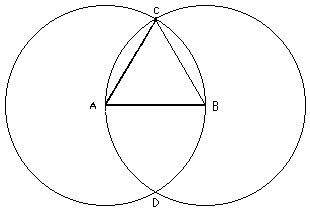To construct an equilateral triangle draw a line segment and mark off the desired length. Set the compass to that length then draw an arc from each end of the given segment so that they intersect at a point on one side. (Again, if in doubt complete circles can be used.) Draw segments connecting each endpoint to the intersection of these segments. Clearly all three sides are of equal length, the triangle is thus equilateral, and since the triangle is isosceles three different ways, by the base angle congruence theorem (yet to come) it is also equiangular.

There are two major ways to construct a regular hexagon. The first method utilizes a circle with radius of the desired hexagon size length. Pick a point on the circle then mark the distance one radii away with your compass. As you procede around the cicle you will make exactly six equally spaced divisions. Connect these in order to complete your hexagon. The second method makes use of the fact that a regular hexagon can be triangulated into six equilateral triangles. Construct an equilateral triangle as above. Draw a circle centered on one vertex and proceed as above.

To construct an angle bisector set the compass to almost any arbitrary but convenient distance. Place the point of the compass at the angle's vertex. Mark auxillary points on each of the angle's rays with the compass at the same distance from the vertex. From each of these two auxillary points draw another arc in the interior of the angle. (A new distance can be selected for these pair, separate from the distance out the arc, but this optional.) The intersection point formed by the arc from each auxillary point are on the line forming the angle bisector. Connect them and extend the line to complete the construction.

### Mazes and Labyrinths

The following link has a maze and a free downloadable Applet to draw perfect mazes: http://www.ericharshbarger.org/java. Perfect mazes are not only solvable, but well-connected and contain no closed loops. Mazes and labyrinths have a long history, probably evolving from defense works against an enemy. Later they evolved into magical devices against evil spirits. Labyrinths often contain blind alleys and are technically different from mazes. In Greek mythology, the Minotaur was confined in the famous labyrinth of Crete. Mazes of hedges or maize (corn) are still common in such gardens as the Versailles, Hampton Court in London, or out in Iowa. Perhaps next year we can take a local field trip to: Wick's Apple House Maize Maze or Barbott Farms. A maze having only one entrance and one exit can be solved by placing one hand against either wall and keeping it there as one traverses the maze. This will usually not be the shortest solution, but the exit can always be reached in this manner. For this to work in a labyrinth, there must be no closed loops. If it has no closed loops it is simply connected otherwise it is multiply connected. Mazes are also used to test learning behavior particularly in mice.

Below/on the next page is a maze from your homework.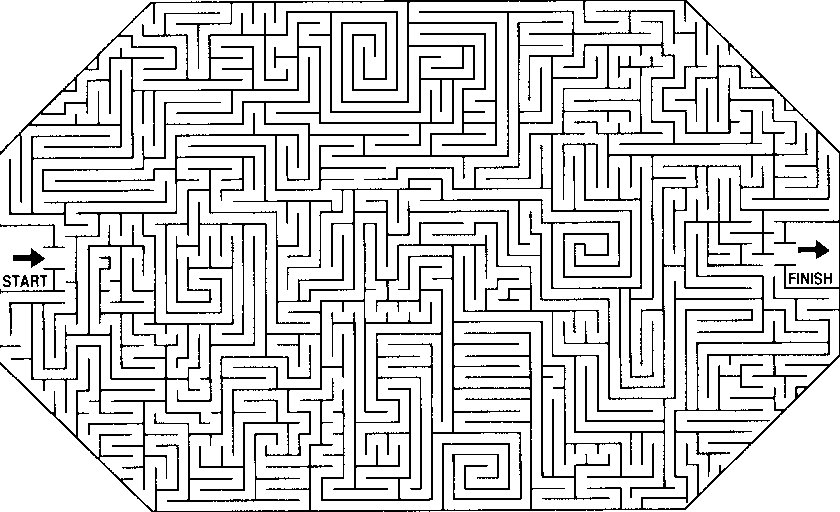For homework 3.7.

BACK HOMEWORK ACTIVITY CONTINUE